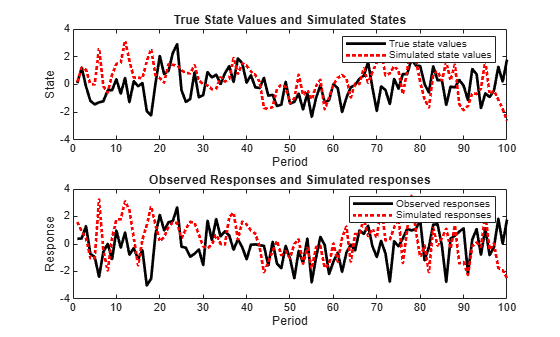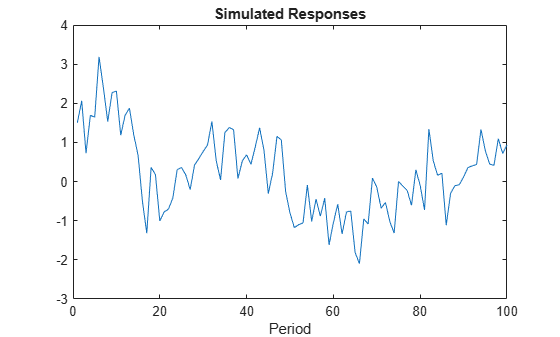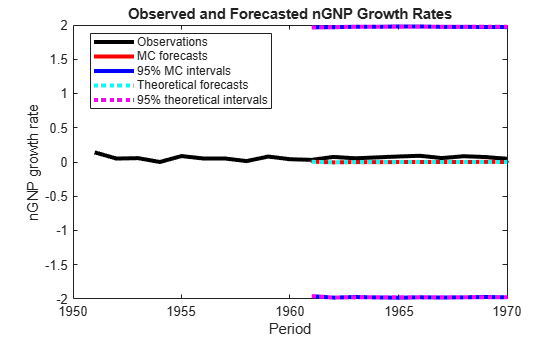# simulate

Class: ssm

Monte Carlo simulation of state-space models

## Syntax

``````[Y,X] = simulate(Mdl,numObs)``````
``````[Y,X] = simulate(Mdl,numObs,Name,Value)``````
``````[Y,X,U,E] = simulate(___)``````

## Description

example

``````[Y,X] = simulate(Mdl,numObs)``` simulates one sample path of observations (`Y`) and states (`X`) from a fully specified, state-space model (`Mdl`). The software simulates `numObs` observations and states per sample path.```
``````[Y,X] = simulate(Mdl,numObs,Name,Value)``` returns simulated responses and states with additional options specified by one or more `Name,Value` pair arguments.For example, specify the number of paths or model parameter values.```

example

``````[Y,X,U,E] = simulate(___)``` additionally simulate state disturbances (`U`) and observation innovations (`E`) using any of the input arguments in the previous syntaxes.```

## Input Arguments

expand all

Standard state-space model, specified as an`ssm` model object returned by `ssm` or `estimate`. A standard state-space model has finite initial state covariance matrix elements. That is, `Mdl` cannot be a `dssm` model object.

If `Mdl` is not fully specified (that is, `Mdl` contains unknown parameters), then specify values for the unknown parameters using the `'``Params``'` `Name,Value` pair argument. Otherwise, the software throws an error.

Number of periods per path to generate variants, specified as a positive integer.

If `Mdl` is a time-varying model, then the length of the cell vector corresponding to the coefficient matrices must be at least `numObs`.

If `numObs` is fewer than the number of periods that `Mdl` can support, then the software only uses the matrices in the first `numObs` cells of the cell vectors corresponding to the coefficient matrices.

Data Types: `double`

### Name-Value Arguments

Specify optional pairs of arguments as `Name1=Value1,...,NameN=ValueN`, where `Name` is the argument name and `Value` is the corresponding value. Name-value arguments must appear after other arguments, but the order of the pairs does not matter.

Before R2021a, use commas to separate each name and value, and enclose `Name` in quotes.

Number of sample paths to generate variants, specified as the comma-separated pair consisting of `'NumPaths'` and a positive integer.

Example: `'NumPaths',1000`

Data Types: `double`

Values for unknown parameters in the state-space model, specified as the comma-separated pair consisting of `'Params'` and a numeric vector.

The elements of `Params` correspond to the unknown parameters in the state-space model matrices `A`, `B`, `C`, and `D`, and, optionally, the initial state mean `Mean0` and covariance matrix `Cov0`.

• If you created `Mdl` explicitly (that is, by specifying the matrices without a parameter-to-matrix mapping function), then the software maps the elements of `Params` to `NaN`s in the state-space model matrices and initial state values. The software searches for `NaN`s column-wise following the order `A`, `B`, `C`, `D`, `Mean0`, and `Cov0`.

• If you created `Mdl` implicitly (that is, by specifying the matrices with a parameter-to-matrix mapping function), then you must set initial parameter values for the state-space model matrices, initial state values, and state types within the parameter-to-matrix mapping function.

If `Mdl` contains unknown parameters, then you must specify their values. Otherwise, the software ignores the value of `Params`.

Data Types: `double`

## Output Arguments

expand all

Simulated observations, returned as a matrix or cell matrix of numeric vectors.

If `Mdl` is a time-invariant model with respect to the observations, then `Y` is a `numObs`-by-n-by-`numPaths` array. That is, each row corresponds to a period, each column corresponds to an observation in the model, and each page corresponds to a sample path. The last row corresponds to the latest simulated observations.

If `Mdl` is a time-varying model with respect to the observations, then `Y` is a `numObs`-by-`numPaths` cell matrix of vectors. `Y{t,j}` contains a vector of length nt of simulated observations for period t of sample path j. The last row of `Y` contains the latest set of simulated observations.

Data Types: `cell` | `double`

Simulated states, returned as a numeric matrix or cell matrix of vectors.

If `Mdl` is a time-invariant model with respect to the states, then `X` is a `numObs`-by-m-by-`numPaths` array. That is, each row corresponds to a period, each column corresponds to a state in the model, and each page corresponds to a sample path. The last row corresponds to the latest simulated states.

If `Mdl` is a time-varying model with respect to the states, then `X` is a `numObs`-by-`numPaths` cell matrix of vectors. `X{t,j}` contains a vector of length mt of simulated states for period t of sample path j. The last row of `X` contains the latest set of simulated states.

Simulated state disturbances, returned as a matrix or cell matrix of vectors.

If `Mdl` is a time-invariant model with respect to the state disturbances, then `U` is a `numObs`-by-h-by-`numPaths` array. That is, each row corresponds to a period, each column corresponds to a state disturbance in the model, and each page corresponds to a sample path. The last row corresponds to the latest simulated state disturbances.

If `Mdl` is a time-varying model with respect to the state disturbances, then `U` is a `numObs`-by-`numPaths` cell matrix of vectors. `U{t,j}` contains a vector of length ht of simulated state disturbances for period t of sample path j. The last row of `U` contains the latest set of simulated state disturbances.

Data Types: `cell` | `double`

Simulated observation innovations, returned as a matrix or cell matrix of numeric vectors.

If `Mdl` is a time-invariant model with respect to the observation innovations, then `E` is a `numObs`-by-h-by-`numPaths` array. That is, each row corresponds to a period, each column corresponds to an observation innovation in the model, and each page corresponds to a sample path. The last row corresponds to the latest simulated observation innovations.

If `Mdl` is a time-varying model with respect to the observation innovations, then `E` is a `numObs`-by-`numPaths` cell matrix of vectors. `E{t,j}` contains a vector of length ht of simulated observation innovations for period t of sample path j. The last row of `E` contains the latest set of simulated observations.

Data Types: `cell` | `double`

## Examples

expand all

Suppose that a latent process is an AR(1) model. The state equation is

`${x}_{t}=0.5{x}_{t-1}+{u}_{t},$`

where ${u}_{t}$ is Gaussian with mean 0 and standard deviation 1.

Generate a random series of 100 observations from ${x}_{t}$, assuming that the series starts at 1.5.

```T = 100; ARMdl = arima('AR',0.5,'Constant',0,'Variance',1); x0 = 1.5; rng(1); % For reproducibility x = simulate(ARMdl,T,'Y0',x0);```

Suppose further that the latent process is subject to additive measurement error. The observation equation is

`${y}_{t}={x}_{t}+{\epsilon }_{t},$`

where ${\epsilon }_{t}$ is Gaussian with mean 0 and standard deviation 0.75. Together, the latent process and observation equations compose a state-space model.

Use the random latent state process (`x`) and the observation equation to generate observations.

`y = x + 0.75*randn(T,1);`

Specify the four coefficient matrices.

```A = 0.5; B = 1; C = 1; D = 0.75;```

Specify the state-space model using the coefficient matrices.

`Mdl = ssm(A,B,C,D)`
```Mdl = State-space model type: ssm State vector length: 1 Observation vector length: 1 State disturbance vector length: 1 Observation innovation vector length: 1 Sample size supported by model: Unlimited State variables: x1, x2,... State disturbances: u1, u2,... Observation series: y1, y2,... Observation innovations: e1, e2,... State equation: x1(t) = (0.50)x1(t-1) + u1(t) Observation equation: y1(t) = x1(t) + (0.75)e1(t) Initial state distribution: Initial state means x1 0 Initial state covariance matrix x1 x1 1.33 State types x1 Stationary ```

`Mdl` is an `ssm` model. Verify that the model is correctly specified using the display in the Command Window. The software infers that the state process is stationary. Subsequently, the software sets the initial state mean and covariance to the mean and variance of the stationary distribution of an AR(1) model.

Simulate one path each of states and observations. Specify that the paths span 100 periods.

`[simY,simX] = simulate(Mdl,100);`

`simY` is a 100-by-1 vector of simulated responses. `simX` is a 100-by-1 vector of simulated states.

Plot the true state values with the simulated states. Also, plot the observed responses with the simulated responses.

```figure subplot(2,1,1) plot(1:T,x,'-k',1:T,simX,':r','LineWidth',2) title({'True State Values and Simulated States'}) xlabel('Period') ylabel('State') legend({'True state values','Simulated state values'}) subplot(2,1,2) plot(1:T,y,'-k',1:T,simY,':r','LineWidth',2) title({'Observed Responses and Simulated responses'}) xlabel('Period') ylabel('Response') legend({'Observed responses','Simulated responses'})```By default, `simulate` simulates one path for each state and observation in the state-space model. To conduct a Monte Carlo study, specify to simulate a large number of paths.

To generate variates from a state-space model, specify values for all unknown parameters.

Explicitly create this state-space model.

`$\begin{array}{c}{x}_{t}=\varphi {x}_{t-1}+{\sigma }_{1}{u}_{t}\\ {y}_{t}={x}_{t}+{\sigma }_{2}{\epsilon }_{t}\end{array}$`

where ${u}_{t}$ and ${\epsilon }_{t}$ are independent Gaussian random variables with mean 0 and variance 1. Suppose that the initial state mean and variance are 1, and that the state is a stationary process.

```A = NaN; B = NaN; C = 1; D = NaN; mean0 = 1; cov0 = 1; stateType = 0; Mdl = ssm(A,B,C,D,'Mean0',mean0,'Cov0',cov0,'StateType',stateType);```

Simulate 100 responses from `Mdl`. Specify that the autoregressive coefficient is 0.75, the state disturbance standard deviation is 0.5, and the observation innovation standard deviation is 0.25.

```params = [0.75 0.5 0.25]; y = simulate(Mdl,100,'Params',params); figure; plot(y); title 'Simulated Responses'; xlabel 'Period';```The software searches for `NaN` values column-wise following the order A, B, C, D, Mean0, and Cov0. The order of the elements in `params` should correspond to this search.

Suppose that the relationship between the change in the unemployment rate (${x}_{1,t}$) and the nominal gross national product (nGNP) growth rate (${x}_{3,t}$) can be expressed in the following, state-space model form.

`$\left[\begin{array}{c}{x}_{1,t}\\ {x}_{2,t}\\ {x}_{3,t}\\ {x}_{4,t}\end{array}\right]=\left[\begin{array}{cccc}{\varphi }_{1}& {\theta }_{1}& {\gamma }_{1}& 0\\ 0& 0& 0& 0\\ {\gamma }_{2}& 0& {\varphi }_{2}& {\theta }_{2}\\ 0& 0& 0& 0\end{array}\right]\left[\begin{array}{c}{x}_{1,t-1}\\ {x}_{2,t-1}\\ {x}_{3,t-1}\\ {x}_{4,t-1}\end{array}\right]+\left[\begin{array}{cc}1& 0\\ 1& 0\\ 0& 1\\ 0& 1\end{array}\right]\left[\begin{array}{c}{u}_{1,t}\\ {u}_{2,t}\end{array}\right]$`

`$\left[\begin{array}{c}{y}_{1,t}\\ {y}_{2,t}\end{array}\right]=\left[\begin{array}{cccc}1& 0& 0& 0\\ 0& 0& 1& 0\end{array}\right]\left[\begin{array}{c}{x}_{1,t}\\ {x}_{2,t}\\ {x}_{3,t}\\ {x}_{4,t}\end{array}\right]+\left[\begin{array}{cc}{\sigma }_{1}& 0\\ 0& {\sigma }_{2}\end{array}\right]\left[\begin{array}{c}{\epsilon }_{1,t}\\ {\epsilon }_{2,t}\end{array}\right],$`

where:

• ${x}_{1,t}$ is the change in the unemployment rate at time t.

• ${x}_{2,t}$ is a dummy state for the MA(1) effect on ${x}_{1,t}$.

• ${x}_{3,t}$ is the nGNP growth rate at time t.

• ${x}_{4,t}$ is a dummy state for the MA(1) effect on ${x}_{3,t}$.

• ${y}_{1,t}$ is the observed change in the unemployment rate.

• ${y}_{2,t}$ is the observed nGNP growth rate.

• ${u}_{1,t}$ and ${u}_{2,t}$ are Gaussian series of state disturbances having mean 0 and standard deviation 1.

• ${\epsilon }_{1,t}$ is the Gaussian series of observation innovations having mean 0 and standard deviation ${\sigma }_{1}$.

• ${\epsilon }_{2,t}$ is the Gaussian series of observation innovations having mean 0 and standard deviation ${\sigma }_{2}$.

Load the Nelson-Plosser data set, which contains the unemployment rate and nGNP series, among other things.

`load Data_NelsonPlosser`

Preprocess the data by taking the natural logarithm of the nGNP series, and the first difference of each. Also, remove the starting `NaN` values from each series.

```isNaN = any(ismissing(DataTable),2); % Flag periods containing NaNs gnpn = DataTable.GNPN(~isNaN); u = DataTable.UR(~isNaN); T = size(gnpn,1); % Sample size y = zeros(T-1,2); % Preallocate y(:,1) = diff(u); y(:,2) = diff(log(gnpn));```

This example proceeds using series without `NaN` values. However, using the Kalman filter framework, the software can accommodate series containing missing values.

To determine how well the model forecasts observations, remove the last 10 observations for comparison.

```numPeriods = 10; % Forecast horizon isY = y(1:end-numPeriods,:); % In-sample observations oosY = y(end-numPeriods+1:end,:); % Out-of-sample observations```

Specify the coefficient matrices.

```A = [NaN NaN NaN 0; 0 0 0 0; NaN 0 NaN NaN; 0 0 0 0]; B = [1 0;1 0 ; 0 1; 0 1]; C = [1 0 0 0; 0 0 1 0]; D = [NaN 0; 0 NaN];```

Specify the state-space model using `ssm`. Verify that the model specification is consistent with the state-space model.

`Mdl = ssm(A,B,C,D)`
```Mdl = State-space model type: ssm State vector length: 4 Observation vector length: 2 State disturbance vector length: 2 Observation innovation vector length: 2 Sample size supported by model: Unlimited Unknown parameters for estimation: 8 State variables: x1, x2,... State disturbances: u1, u2,... Observation series: y1, y2,... Observation innovations: e1, e2,... Unknown parameters: c1, c2,... State equations: x1(t) = (c1)x1(t-1) + (c3)x2(t-1) + (c4)x3(t-1) + u1(t) x2(t) = u1(t) x3(t) = (c2)x1(t-1) + (c5)x3(t-1) + (c6)x4(t-1) + u2(t) x4(t) = u2(t) Observation equations: y1(t) = x1(t) + (c7)e1(t) y2(t) = x3(t) + (c8)e2(t) Initial state distribution: Initial state means are not specified. Initial state covariance matrix is not specified. State types are not specified. ```

Estimate the model parameters, and use a random set of initial parameter values for optimization. Restrict the estimate of ${\sigma }_{1}$ and ${\sigma }_{2}$ to all positive, real numbers using the `'lb'` name-value pair argument. For numerical stability, specify the Hessian when the software computes the parameter covariance matrix, using the `'CovMethod'` name-value pair argument.

```rng(1); params0 = rand(8,1); [EstMdl,estParams] = estimate(Mdl,isY,params0,... 'lb',[-Inf -Inf -Inf -Inf -Inf -Inf 0 0],'CovMethod','hessian');```
```Method: Maximum likelihood (fmincon) Sample size: 51 Logarithmic likelihood: -170.92 Akaike info criterion: 357.84 Bayesian info criterion: 373.295 | Coeff Std Err t Stat Prob ---------------------------------------------------- c(1) | 0.06750 0.16548 0.40791 0.68334 c(2) | -0.01372 0.05887 -0.23302 0.81575 c(3) | 2.71201 0.27039 10.03006 0 c(4) | 0.83816 2.84586 0.29452 0.76836 c(5) | 0.06274 2.83470 0.02213 0.98234 c(6) | 0.05196 2.56873 0.02023 0.98386 c(7) | 0.00272 2.40771 0.00113 0.99910 c(8) | 0.00016 0.13942 0.00113 0.99910 | | Final State Std Dev t Stat Prob x(1) | -0.00000 0.00272 -0.00033 0.99973 x(2) | 0.12237 0.92954 0.13164 0.89527 x(3) | 0.04049 0.00016 256.77783 0 x(4) | 0.01183 0.00016 72.52162 0 ```

`EstMdl` is an `ssm` model, and you can access its properties using dot notation.

Filter the estimated, state-space model, and extract the filtered states and their variances from the final period.

`[~,~,Output] = filter(EstMdl,isY);`

Modify the estimated, state-space model so that the initial state means and covariances are the filtered states and their covariances of the final period. This sets up simulation over the forecast horizon.

```EstMdl1 = EstMdl; EstMdl1.Mean0 = Output(end).FilteredStates; EstMdl1.Cov0 = Output(end).FilteredStatesCov;```

Simulate `5e5` paths of observations from the fitted, state-space model `EstMdl`. Specify to simulate observations for each period.

```numPaths = 5e5; SimY = simulate(EstMdl1,10,'NumPaths',numPaths);```

`SimY` is a `10`-by- `2`-by- `numPaths` array containing the simulated observations. The rows of `SimY` correspond to periods, the columns correspond to an observation in the model, and the pages correspond to paths.

Estimate the forecasted observations and their 95% confidence intervals in the forecast horizon.

```MCFY = mean(SimY,3); CIFY = quantile(SimY,[0.025 0.975],3);```

Estimate the theoretical forecast bands.

```[Y,YMSE] = forecast(EstMdl,10,isY); Lb = Y - sqrt(YMSE)*1.96; Ub = Y + sqrt(YMSE)*1.96;```

Plot the forecasted observations with their true values and the forecast intervals.

```figure h = plot(dates(end-numPeriods-9:end),[isY(end-9:end,1);oosY(:,1)],'-k',... dates(end-numPeriods+1:end),MCFY(end-numPeriods+1:end,1),'.-r',... dates(end-numPeriods+1:end),CIFY(end-numPeriods+1:end,1,1),'-b',... dates(end-numPeriods+1:end),CIFY(end-numPeriods+1:end,1,2),'-b',... dates(end-numPeriods+1:end),Y(:,1),':c',... dates(end-numPeriods+1:end),Lb(:,1),':m',... dates(end-numPeriods+1:end),Ub(:,1),':m',... 'LineWidth',3); xlabel('Period') ylabel('Change in the unemployment rate') legend(h([1,2,4:6]),{'Observations','MC forecasts',... '95% forecast intervals','Theoretical forecasts',... '95% theoretical intervals'},'Location','Best') title('Observed and Forecasted Changes in the Unemployment Rate')``````figure h = plot(dates(end-numPeriods-9:end),[isY(end-9:end,2);oosY(:,2)],'-k',... dates(end-numPeriods+1:end),MCFY(end-numPeriods+1:end,2),'.-r',... dates(end-numPeriods+1:end),CIFY(end-numPeriods+1:end,2,1),'-b',... dates(end-numPeriods+1:end),CIFY(end-numPeriods+1:end,2,2),'-b',... dates(end-numPeriods+1:end),Y(:,2),':c',... dates(end-numPeriods+1:end),Lb(:,2),':m',... dates(end-numPeriods+1:end),Ub(:,2),':m',... 'LineWidth',3); xlabel('Period') ylabel('nGNP growth rate') legend(h([1,2,4:6]),{'Observations','MC forecasts',... '95% MC intervals','Theoretical forecasts','95% theoretical intervals'},... 'Location','Best') title('Observed and Forecasted nGNP Growth Rates')```## Tips

Simulate states from their joint conditional posterior distribution given the responses by using `simsmooth`.

 Durbin J., and S. J. Koopman. Time Series Analysis by State Space Methods. 2nd ed. Oxford: Oxford University Press, 2012.